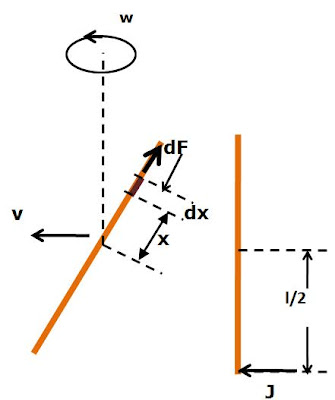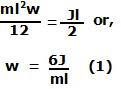## Friday, February 13, 2009

### Irodov Problem 1.273Impulse is a force that acts for a very short duration and cause momentum to change almost instantaneously. The change in momentum of the rod will thus be J. In other words, the CG of the rod will start to move at a speed v=J/m.

The impulse also imparts an impulsive angular momentum of Jl/2 about an axis perpendicular to the plane of the rod passing through its CG (since it acts at a distance l/2 to the CG) to the rod sending it into a spin. Let the final angular velocity of the rod about its CG be w. Then we have,Consider an infinitesimally small piece of the rod of length dx at a distance x from the axis along the length of the rod. If the linear density (mass per unit length) of the rod is p, then the mass of this piece is pdx. The rod spins about the CG at a rate w and so it exerts a centrifugal force of. The net force exterted by one half of the rod on the other is the integration of dF over the half length of the rod as,